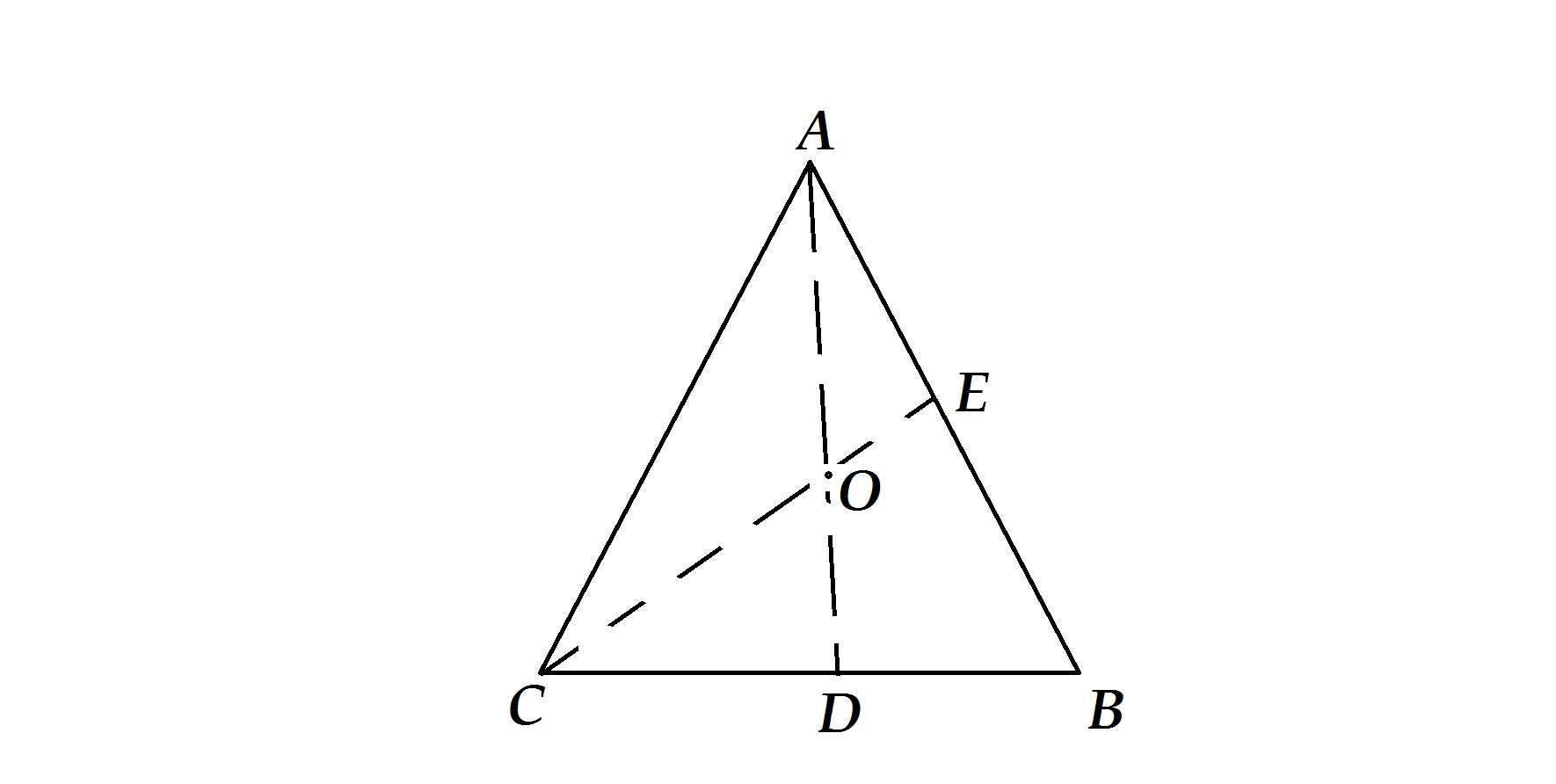# Is the Given Information Sufficient?

Geometry Level 4Let $O$ be a point inside $\triangle ABC$ such that

$\dfrac{OC}{OE}=\frac{10}{3} ~~~\text{and} ~~~\dfrac{OA}{OD}=\frac{9}{4}.$

If

$\dfrac{BE}{EA}+\dfrac{BD}{DC}=\dfrac{m}{n},$

where $\gcd(m,n)=1$, find the value of $m+n-2$.

×